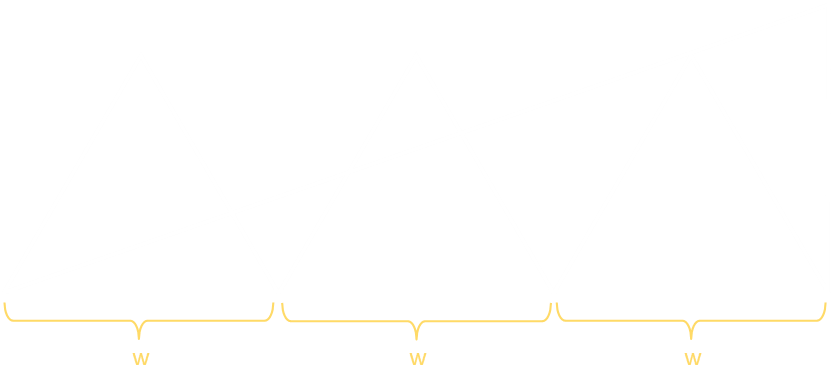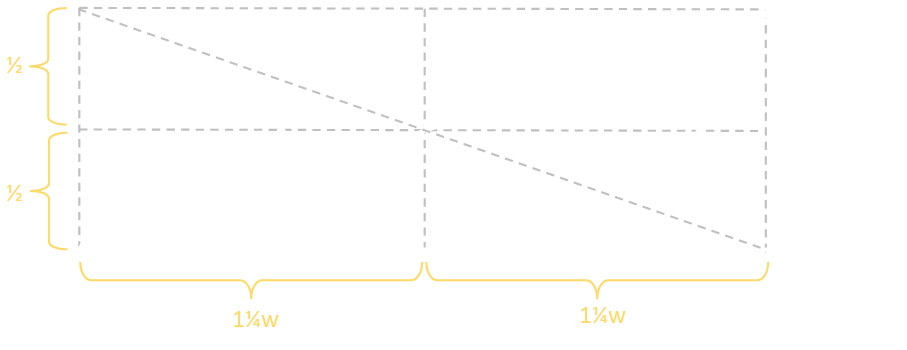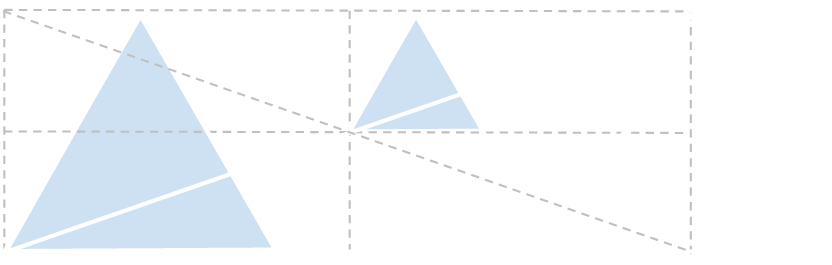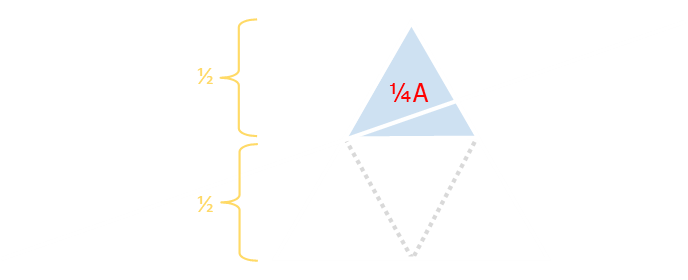# Three Triangles Puzzle

A quick little puzzle this week. (I tried to track down the original source, but reached a dead-end with a web search as the site that hosted it, a blogspot page under the name fivetriangles appears password protected, and no longer maintained). Anyway, here's the problem, and my (roundabout) way of solving it:There are three identical triangles with aligned bases (in the original problem, it is stated they are equilateral, but I don't think that really matters; Any congruent triangles will do, and I'm going to use isoceles triangles in my solving). If we say that one triangle has the area A, what is the area of the two shaded regions?
What is the area of the shaded regions?

## Solving

I wanted to see if I could solve the problem using just similar triangles, and without using trigonometry.Let's call the base of each triangle w, and extend the diagonal line further to align with the edge of the third triangle.We can see the extended line continues on another ½w, and we'll normalize the height of the triangles to be 1.We can draw two similar triangles, and this allows us to calculate the height h:
h / 3w = 1 / 2½w
h = 6/5We can see three other congruent triangles which are similar to the larger triangle. Each of these small triangles is of height ⅓h.Each of these little triangles is a scaled down version of the big triangles, and so we can see that x, is ⅙w (one sixth of the width of one of the triangles; this makes sense as the difference between the two large triangles is ½w, and this is equally divided into three). This gives w-x = ⅚w.We need to calculate the altitude y of the red triangle. Again from similar triangles:
6y/5w = h/3w
y = 5h/18
But we already calculated h=6/5, so this gives the result that y=1/3.We know the area of one triangle is A, and this is with an altitude of 1, and so the area of any triangle with the same base and altitude 1/3 (middle diagram above), will just be A/3. The complement of this is the triangle we want, and so this works out to be 2A/3. We are halfway there.
To work on the second triangular area, we revert back to the bigger picture and put a rectangle around the the two and half triangles and we can draw in the opposite diagonal. These cross in the middle (you can also convince yourself of this from rotational symmetery).Again we see similar triangles. The top of the middle triangle is just a scaled down version of the left triangle.Since it is half the altitude and with half the base, then it has a quarter of the area A.From the similar nature, this quarter sized triangle will have a quarter of the area of his big brother; ¼ × ⅔A = ⅙A.

## Result

Putting this all together the two shaded regions = ⅔A + ⅙A = ⅚A.